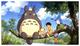• # Help for Solve Sort by Extensions Problem

This is my solution code:

```def sort_by_ext (files: List[str]) -> List[str]:

# Preprocessing
files_replace = [i.replace(" ", "") for i in files]
files_split = [i.split(".") for i in files_replace]
print(f'files_split: {files_split}')

#Case files without extension
without_extention = sorted([i for i in files_split if len(i) == 1])
print(f'without_extention: {without_extention}')

# Case "normal"
ext_files = filter(lambda elements: len(elements) == 2 and elements != '', files_split)
files1 = sorted(ext_files, key=lambda ele: ele, reverse = False)
ext_sorted = [ ".".join(i) for i in files1]
print(f'ext_sorted: {ext_sorted}')

# With two "."
two_extensions = (filter(lambda element:len(element)>=3, files_split ))
files3 = sorted(two_extensions, key = lambda ele: ele, reverse = False)
two_sorted = [".".join(i) for i in files3]
print(f'two_extensions: {two_sorted}')

#Case files that are only extension
only_ext_files = filter(lambda elements: len(elements) == 2 and elements == '', files_split)
files2 = sorted(only_ext_files, key=lambda ele: ele, reverse = False)
only_ext_sorted = [ ".".join(i) for i in files2]
print(f'only_ext_sorted: {only_ext_sorted}')

#all in good order
final = without_extention + only_ext_sorted + ext_sorted + two_sorted
print(f'final: {final}')
return final
```

This method cannot process problems like this ([".config","my.doc","1.exe","345.bin","green.bat","format.c","no.name.","best.test.exe"])

The right result should be: [".config","no.name.","green.bat","345.bin","format.c","my.doc","1.exe","best.test.exe"]

But mine was: [".config","green.bat","345.bin","format.c","my.doc","1.exe","no.name.","best.test.exe"]

Thanks for your time and help!5